Compound Interest

# Compound Interest Notes | Study Quantitative Aptitude for Competitive Examinations - Banking Exams

## Document Description: Compound Interest for Banking Exams 2022 is part of Quantitative Aptitude for Competitive Examinations preparation. The notes and questions for Compound Interest have been prepared according to the Banking Exams exam syllabus. Information about Compound Interest covers topics like and Compound Interest Example, for Banking Exams 2022 Exam. Find important definitions, questions, notes, meanings, examples, exercises and tests below for Compound Interest.

Introduction of Compound Interest in English is available as part of our Quantitative Aptitude for Competitive Examinations for Banking Exams & Compound Interest in Hindi for Quantitative Aptitude for Competitive Examinations course. Download more important topics related with notes, lectures and mock test series for Banking Exams Exam by signing up for free. Banking Exams: Compound Interest Notes | Study Quantitative Aptitude for Competitive Examinations - Banking Exams
 1 Crore+ students have signed up on EduRev. Have you?

Compound Interest

The difference between the amount and the money borrowed is called the compound interest for given period of time
1) Let principal =P; time =n years; and rate = r% per annum and let A be the total amount at the end of n years, then
A = P*[1+ (r/100)]n;
CI = {P*[1+ (r/100)]n -1}
2) When compound interest reckoned half yearly, then r% become r/2% and time n become 2n;
A= P*[1+ (r/2*100)]2n
3) For quarterly
A= P*[1+ (r/4*100)]4n
4) The difference between compound interest and simple interest over two years is given by
Pr2/1002or P(r/100)2
5) The difference between compound interest and simple interest over three years is given by
P(r/100)2*{(r/100)+3}
6) When Rates are different for different years, say R1%, R2%, R3% for 1st, 2nd and 3rd year respectively, Then total amount is given by
P ((1 + R1)/100) ((1 + R2)/100) ((1 + R2)/100)
7) Present worth of Rs. x due n years hence is given by
x/(1+R/100)

1). Interest is compounded half-yearly, therefore,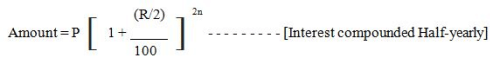Example:
Find the compound interest on Rs. 20,000 in 2 years at 4 % per annum, the interest being compounded half-yearly.
Solution:

Principal = Rs. 20000, Rate = 2 % per half-year, Time = 2 years = 4 half- years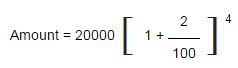Amount=Rs.21648.64

Compound Interest = Total amount – Principal
= 21648.64 – 20000
= Rs. 1648.64

2). If interest is compounded annually,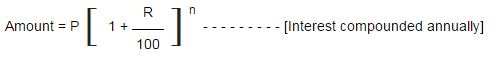Example:
Find compound interest on Rs. 8500 at 4 % per annum for 2 years, compounded annually.
Solution:

We are given:
Principal = Rs. 8500, Rate = 4 % per annum, Time = 2 years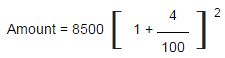= Rs. 9193.6
Compound Interest = Total amount – Principal
= 9193.6 – 8500
= 693.6
Compound Interest = Rs. 693.6

3). When Rates are different for different years, say R1%, R2%, R3% for 1st, 2nd and 3rd year respectively. Then, Amount (= Principal + Compound interest) = P(1 + R1/100)(1 + R2/100)(1 + R3/100).
Example:
Find the compound interest on a principal amount of Rs.5000 after 2 years, if the rate of interest for the 1st year is 2% and for the 2nd year is 4%.
Solution:
Here R1 = 2% R2 = 4% and p = Rs.5000, we have to find CI (compound interest).
CI = 5000(1 + 2/100)(1 + 4/100) – 5000
= 5000 x (102/100)(104/100) – 5000
= 5000 x (51/50) x (52/50) – 5000
= 5000 x (51 x 52/2500) – 5000
= 5000 x (2652 / 2500) – 5000
= 5304 – 5000 = 304
Hence the required compound interest is Rs.304.

4). When compound interest is reckoned half-yearly.
If the annual rate is r% per annum and is to be calculated for n years, then in this case, rate = (n/2%) half-yearly and time = (2n) half-yearly.

Form the above we get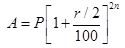Example:
Sam investment Rs.15,000 @ 10% per annum for one year. If the interest is compounded half-yearly, then the amount received by Sam at the end of the year will be.
Solution:
P = Rs. 15000; R = 10% p.a = 5% half-year, T = 1 year = 2 half year

Amount = Rs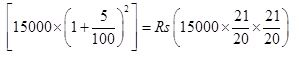= Rs.16537.50

If the simple interest for certain sum for 2yrs at the annual rate of interest R% is SI. Then,
Compound interest (CI) = SI (1+r/200)   (no. of years =2)

5). If the simple interest for a certain sum for 2 yr at 5%pa is 200, then what will be the compound interest for same sum for same period and the same rate of interest?

Sol:
Si =200 r=5%
Ci =200(1+5/200) =200*(205/200) =205
If a certain sum at compound interest becomes x times n1^yr and y times n2^yr then,
X1/N1 = Y1/N2

6). If an amount at compound interest becomes twice in 5yr, then in how many years, it will be 16 times at the same rate of interest?
21/5  = 16 1/x2
=24*1/x2
1/5 = 4/x2
X2 = 5*4 =20yrs
If a certain sum at compound interest amounts to A1 in   n yrs and A2 in (n+1) yrs,
then
Rate of compound interest =(A2 – A1)/A1 *100%
Sum = A1 (A1 /A2)n

7).  A sum of money invested at compound interest amounts to 800 in 2yr and 840 in 3yrs .Find the rate of interest and the sum.

A1 =800 ; A=840,
Rate of interest = (840-800)/800 *100% =40/8 =5%
Sum = 800 *(800/840)2 =320000/441 = Rs.725.62
If the populations of a city P and it increases with the rate of R% per annum, then

• Populations after n yr = p(1+R/100)n
• Populations n yr ago = p / (1+R/100)n

8). The population of a city A is 5000. It increases by 10% in 1st year. It decreases by 20% in the 2nd yr because of some reason. In the 3rd yr, the population increases by 30%. What will be the [population of area A at the end of 3yrs?
=5000(1+10/100)(1-20/100)(1+30/100)
= 500*(11/10)*(4/5)*(13/10) = 5720
Difference between ci and si 2yr =pr2 /1002

9). The difference between c.i and s.i for 2yr at the rate of 5% per annum is 5 .then the sum
5 = p (5/100)2 = Rs.2000
Rate of interest (no .of years =2)
(for only ci)
2% = 4.04%
3% = 6.09%
4% = 8. 16%
5% = 10.25%
6% = 12.36%
7%   = 14.49%
8% = 16.64%
9% = 18.81%
10%= 20.00
+ 1.00 =21%

10). What is the Compound interest for Rs. 1500 at 5% rate of interest for 2 years?
1500*(10.25/100) =153.75

### Difference between the compound interest and the simple interest

Example:
If the difference between the compound interest and the simple interest on a certain sum of money at 5% per annum for 3 years is Rs. 1220. What is the sum?

Solution: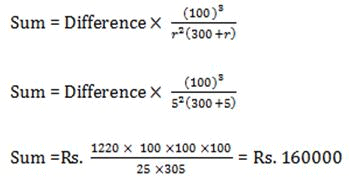The document Compound Interest Notes | Study Quantitative Aptitude for Competitive Examinations - Banking Exams is a part of the Banking Exams Course Quantitative Aptitude for Competitive Examinations.
All you need of Banking Exams at this link: Banking Exams

## Quantitative Aptitude for Competitive Examinations

33 videos|34 docs|140 tests
 Use Code STAYHOME200 and get INR 200 additional OFF

## Quantitative Aptitude for Competitive Examinations

33 videos|34 docs|140 tests

Track your progress, build streaks, highlight & save important lessons and more!

,

,

,

,

,

,

,

,

,

,

,

,

,

,

,

,

,

,

,

,

,

;